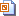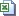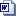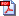Nuffield Mathematics teaching resources are for use in secondary and further education

# Level 3 Algebra

## Maximum and minimum problems

Using graphical methods to solve maximum and minimum problems in industry and in working life, using a spreadsheet or graphic calculator.

## Climate prediction

There are two versions of this activity.

• Students create (A) spreadsheets or use
• (B) graphic calculators to model what would happen to the temperature of the Earth if there were to be a sudden change in the amount of radiation entering or leaving the planet. Then they investigate polynomial and exponential functions to find the best model.

## Gas guzzlers

Students use graphical methods to find a suitable model to connect two sets of data. Students can use their model(s) to make predictions. They should also be encouraged to work out percentage errors to test and compare the accuracy of their model(s).

## Coughs and sneezes

Students use polynomial and trigonometric functions to model the number of people suffering from a cold over several weeks.

## Cup of coffee

Students model data from measuring how long caffeine from tea, coffee or cola stays in the human body’s bloodstream. This involves drawing graphs, then finding functions and parameters to fit different models to the graphs.

## How frequent are earthquakes?

Students use exponential and logarithmic functions (and linear graphs) to explore earthquake data and to model a relationship between the magnitude of earthquakes and how frequently they occur.

## Smoke strata

Practice in using logarithmic graphs to check that a power law is a good model for data and find the associated parameters.

## Water flow

Students use linear and quadratic functions to model data, and calculate percentage errors.

## Test run

Students interpret a speed–time graph for a car during a test run, and fit linear and quadratic models to the graph.Slides 2007 (1.6 MB)Teacher presentation (1.3 MB)Student data spreadsheet (226 KB)Student sheets Word 2007 (873 KB)Student sheets pdf (308 KB)Teacher notes Word (164 KB)Teacher notes pdf (383 KB)

## Completing the square

Students match cards to practise completing the square and relating the competed square form to the graph of the function.

## Ozone hole

Students suggest types of function to model ozone hole data (given in a table and also a graph) before using a graphic calculator or spreadsheet to find at least two particular models. They then consider how well their functions model the data and what they predict for the future.Slides (1.3 MB)Student sheets 2007 Word (376 KB)Student sheets pdf (364 KB)Teacher notes Word (162 KB)Teacher notes pdf (299 KB)